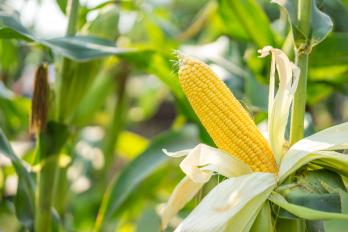# MAKING A GOOD ESTIMATE ON YIELDS

#### Aug 23, 2021#### Agronomy

Is it possible to estimate yield? Sure. It’s a pretty simple process, according to Bob Marquette, Federated agronomist at the Albertville location. In essence, “you measure the fields, go pick some ears [around the field], do some counting and do some math . . . and there you go!”

But is this estimation accurate? Again, yes, within 20 bu./ac. +/- (the weigh wagon and/or  yield monitor will determine the final numbers).

Today the ears of corn are well established and drying down; “drying is happening way too fast this year,” said Marquette, due to the season’s high temps and minimal rain.

Follow this link for more complete details, as provided by Bayer®, but here is a summary of the process:

##### Yield Component Method

This method can be used as early as the milk growth stage (R3).

1. Identify several sample areas (1/1000th of an acre each) and collect one fully filled ear of corn from each area.
2. Count the kernels on each ear where there are complete rings of kernels around the ear (skip the tip and butt of the ear).
3. Tally the number of kernel rows per year on 1 of every 5 ears, and compute the average.
4. On those same ears, count the number of kernels per row, and compute the average.
5. The yield per acre is then computed by multiplying the number of ears by the average number of rows by the average number of kernels, divided by 90. The divisor, 90 in a very good year, should be determined by the growing conditions. See chart below.
6. Complete these steps across multiple sample sites in the field; then calculate the average to estimate yield potential across the whole field.
 Growing conditions Factor Range in kernel number/bu. Excellent 75-80 75,000 to 80,000 Average 85-90 85,000 to 90,000 Poor 95-105 95,000 to 105,000
##### Ear Weight Method

This method can only be used when corn has reached the black layer stage (R6).

First, Identify several sample areas (1/1000th of an acre each) and count the number of harvestable ears in each area. See chart below.

 Row width  (in inches) Row length  (for 1/1000th of an acre) 20” 26’2” 22” 23’9” 30” 17’5” 36” 14’6” 38” 13”9”

Then, weigh 1 of every 5 ears and calculate the average ear weight per area; hand shell the kernels and measure grain moisture with a tester.

• - Calculate the average yield potential in each area:
• - Multiply the number of ears by the average ear weight.
• - Multiply average grain moisture by 1.1411.
• - Add 46.2 to the result of (b).
• - Divide the result of (a) by the result of (c).
• - Multiply the result of (d) by 1000.

In the end, harvest is the only fully accurate determiner of yields, but these methods can help with planning for harvest and next growing season. Talk to your Federated Agronomist with any questions.